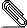# [Hol-info] An infinite sum of 0 or 1 is finite, then ...

```Hi,

I'm blocked at the following goal:```
```
I have a function f returning either 0 or 1.  Now I know the infinite
sum of f is finite, i.e.

suminf f < PosInf       (or `summable f` speaking reals)

How can I prove the set of {x | f x = 1} is finite, or after certain
index m all the rest f(n) are zeros?

∃m. ∀n. m ≤ n ⇒ (f n = 0)

If I use CCONTR_TAC (proof by contradiction), I can easily derive the
following 2 assumptions using results I established in my previous
similar questions:

INFINITE N
∀n. n ∈ N ⇒ (f n = 1)

but still I've no idea how to derive a contradiction with `suminf f <
PosInf` by proving `suminf f = PosInf`...

Thanks,

Chun Tian

```signature.asc
Description: OpenPGP digital signature

```_______________________________________________
hol-info mailing list
hol-info@lists.sourceforge.net
https://lists.sourceforge.net/lists/listinfo/hol-info
```